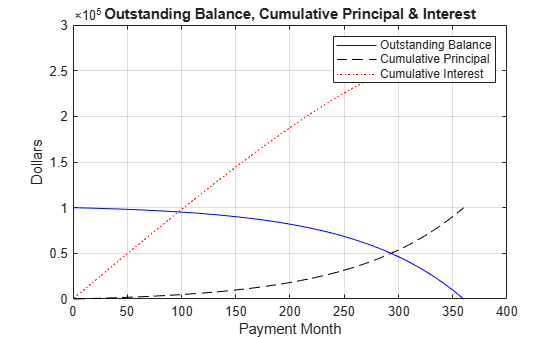# amortize

Amortization schedule

## Syntax

``[Principal,Interest,Balance,Payment] = amortize(Rate,NumPeriods,PresentValue)``
``[Principal,Interest,Balance,Payment] = amortize(___,FutureValue,Due)``

## Description

example

````[Principal,Interest,Balance,Payment] = amortize(Rate,NumPeriods,PresentValue)` returns the principal and interest payments of a loan, the remaining balance of the original loan amount, and the periodic payment.```

example

````[Principal,Interest,Balance,Payment] = amortize(___,FutureValue,Due)` specifies options using one or more optional arguments in addition to the input arguments in the previous syntax.```

## Examples

collapse all

Compute an amortization schedule for a conventional 30-year, fixed-rate mortgage with fixed monthly payments and assume a fixed rate of 12% APR and an initial loan amount of \$100,000.

```Rate = 0.12/12; % 12 percent APR = 1 percent per month NumPeriods = 30*12; % 30 years = 360 months PresentValue = 100000; [Principal, Interest, Balance, Payment] = amortize(Rate, ... NumPeriods, PresentValue);```

The output argument `Payment` contains the fixed monthly payment.

```format bank Payment```
```Payment = 1028.61 ```

Summarize the amortization schedule graphically by plotting the current outstanding loan balance, the cumulative principal, and the interest payments over the life of the mortgage. In particular, note that total interest paid over the life of the mortgage exceeds \$270,000, far in excess of the original loan amount.

```plot(Balance,'b'), hold('on') plot(cumsum(Principal),'--k') plot(cumsum(Interest),':r') xlabel('Payment Month') ylabel('Dollars') grid('on') title('Outstanding Balance, Cumulative Principal & Interest') legend('Outstanding Balance', 'Cumulative Principal', ... 'Cumulative Interest')```The solid blue line represents the declining principal over the 30-year period. The dotted red line indicates the increasing cumulative interest payments. Finally, the dashed black line represents the cumulative principal payments, reaching \$100,000 after 30 years.

## Input Arguments

collapse all

Interest-rate per period, specified as a scalar numeric decimal.

Data Types: `double`

Number of payment periods, specified as a scalar numeric.

Data Types: `double`

Present value of the loan, specified as a scalar numeric.

Data Types: `double`

(Optional) Future value of the loan, specified as a scalar numeric.

Data Types: `double`

(Optional) When payments are due, specified as a scalar integer with value of `0` (end of period) or `1` (beginning of period).

Data Types: `double`

## Output Arguments

collapse all

Principal paid in each period, returned as a `1`-by-`NumPeriods` vector.

Interest paid in each period, returned as a `1`-by-`NumPeriods` vector.

Remaining balance of the loan in each payment period, returned as a `1`-by-`NumPeriods` vector.

Payment per period, returned as a scalar numeric.

## Version History

Introduced before R2006a